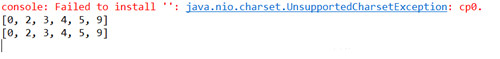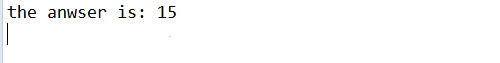# java架构如何嵌入python？Java调用Python程序方法

1. 在java类中直接执行python语句

· Jython是一种完整的语言，而不是一个Java翻译器或仅仅是一个Python编译器，它是一个Python语言在Java中的完全实现。Jython也有很多从CPython中继承的模块库。最有趣的事情是Jython不像CPython或其他任何高级语言，它提供了对其实现语言的一切存取。所以Jython不仅给你提供了Python的库，同时也提供了所有的Java类。这使其有一个巨大的资源库。

```<dependency >
.
. <
groupId > org.python < /groupId>
.
. <
artifactId > jython - standalone < /artifactId>
.
. <
version > 2.7 .0 < /version>
.
. <
/dependency>```

```PythonInterpreter interpreter = new PythonInterpreter();
interpreter.exec("a=[5,2,3,9,4,0]; ");
interpreter.exec("print(sorted(a));"); //此处python语句是3.x版本的语法
interpreter.exec("print sorted(a);"); //此处是python语句是2.x版本的语法```2. 在java中调用本地python脚本

```def add(a,b):
return a + b```

python的功能函数已经写好，接下来我们写一个java的测试类(同样需要用到Jpython包)，来测试一下是否可以运行成功。代码如下：

```import org.python.core.PyFunction;
import org.python.core.PyInteger;
import org.python.core.PyObject;
import org.python.util.PythonInterpreter;
public class Java_Python_test
{
public static void main(String[] args)
{
// TODO Auto-generated method stub
PythonInterpreter interpreter = new PythonInterpreter();
// 第一个参数为期望获得的函数(变量)的名字，第二个参数为期望返回的对象类型
int a = 5, b = 10;
//调用函数，如果函数需要参数，在Java中必须先将参数转化为对应的“Python类型”
PyObject pyobj = pyFunction.__call__(new PyInteger(a), new PyInteger(b));
System.out.println("the anwser is: " + pyobj);
}
}```3. 使用Runtime.getRuntime()执行脚本文件(推荐)

```import numpy as np
a = np.arange(12).reshape(3,4)
print(a)```

```import java.io.BufferedReader;
import java.io.IOException;
public class Demo1
{
public static void main(String[] args)
{
// TODO Auto-generated method stub
Process proc;
try
{
proc = Runtime.getRuntime()
.exec("python D:\\demo1.py"); // 执行py文件
//用输入输出流来截取结果
String line = null;
while ((line = in .readLine()) != null)
{
System.out.println(line);
} in .close();
proc.waitFor();
}
catch (IOException e)
{
e.printStackTrace();
}
catch (InterruptedException e)
{
e.printStackTrace();
}
}
}```java开发框架有哪些?java开发框架介绍

java软件怎么改中文?Java有哪些好用的软件?

java程序怎么输出一串整数?java输出方式有哪些?